Next: Eigenvalues of Up: Orbital Angular Momentum Previous: Eigenstates of Angular Momentum

# Eigenvalues ofIt seems reasonable to attempt to write the eigenstate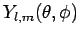in the separable form(570)

We can satisfy the orthonormality constraint (558) provided that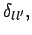(571)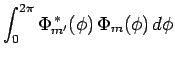(572)

Note, from Eq. (553), that the differential operator which representsonly depends on the azimuthal angle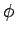, and is independent of the polar angle. It therefore follows from Eqs. (553), (556), and (570) that(573)

The solution to this equation is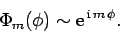(574)

Here, the symboljust means that we are neglecting multiplicative constants.

Now, our basic interpretation of a wavefunction as a quantity whose modulus squared represents the probability density of finding a particle at a particular point in space suggests that a physical wavefunction must be single-valued in space. Otherwise, the probability density at a given point would not, in general, have a unique value, which does not make physical sense. Hence, we demand that the wavefunction (574) be single-valued: i.e.,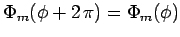for all. This immediately implies that the quantityis quantized. In fact,can only take integer values. Thus, we conclude that the eigenvalues ofare also quantized, and take the values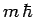, whereis an integer. [A more rigorous argument is that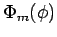must be continuous in order to ensure thatis an Hermitian operator, since the proof of hermiticity involves an integration by parts inthat has canceling contributions from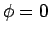and.]

Finally, we can easily normalize the eigenstate (574) by making use of the orthonormality constraint (572). We obtain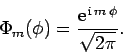(575)

This is the properly normalized eigenstate ofcorresponding to the eigenvalue.Next: Eigenvalues of Up: Orbital Angular Momentum Previous: Eigenstates of Angular Momentum
Richard Fitzpatrick 2010-07-20